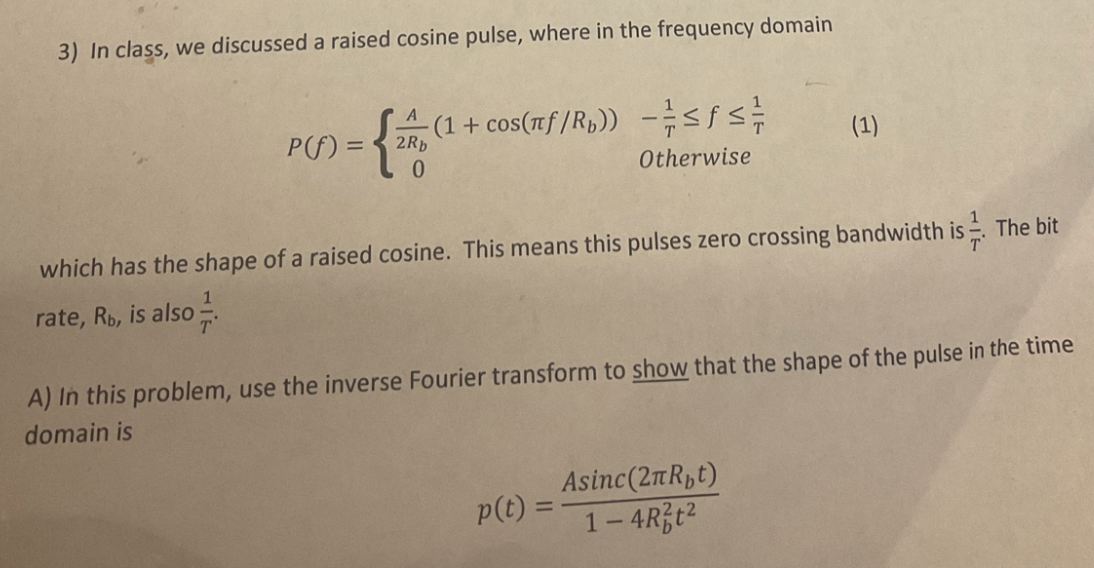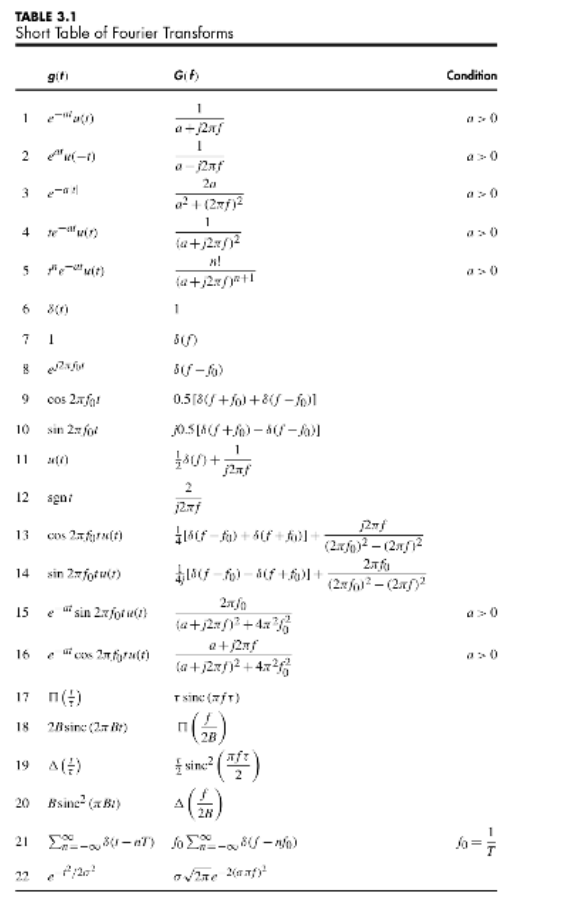# (Solved): Fourier Transform with Digital and analog communication system problem Please answer all of the ques ...

Fourier Transform with Digital and analog communication system problem

I suck at this stuff...3) In class, we discussed a raised cosine pulse, where in the frequency domain $P(f)=\left\{\begin{array}{ll} \frac{A}{2 R_{b}}\left(1+\cos \left(\pi f / R_{b}\right)\right) & -\frac{1}{T} \leq f \leq \frac{1}{T} \\ 0 & \text { Otherwise } \end{array}\right.$ which has the shape of a raised cosine. This means this pulses zero crossing bandwidth is $$\frac{1}{T}$$. The bit rate, $$\mathrm{R}_{\mathrm{b}}$$, is also $$\frac{1}{T}$$ A) In this problem, use the inverse Fourier transform to show that the shape of the pulse in the time domain is $p(t)=\frac{A \operatorname{sinc}\left(2 \pi R_{b} t\right)}{1-4 R_{b}^{2} t^{2}}$ TABLE $$3.1$$ Short Table of Fourier Transforms

We have an Answer from Expert